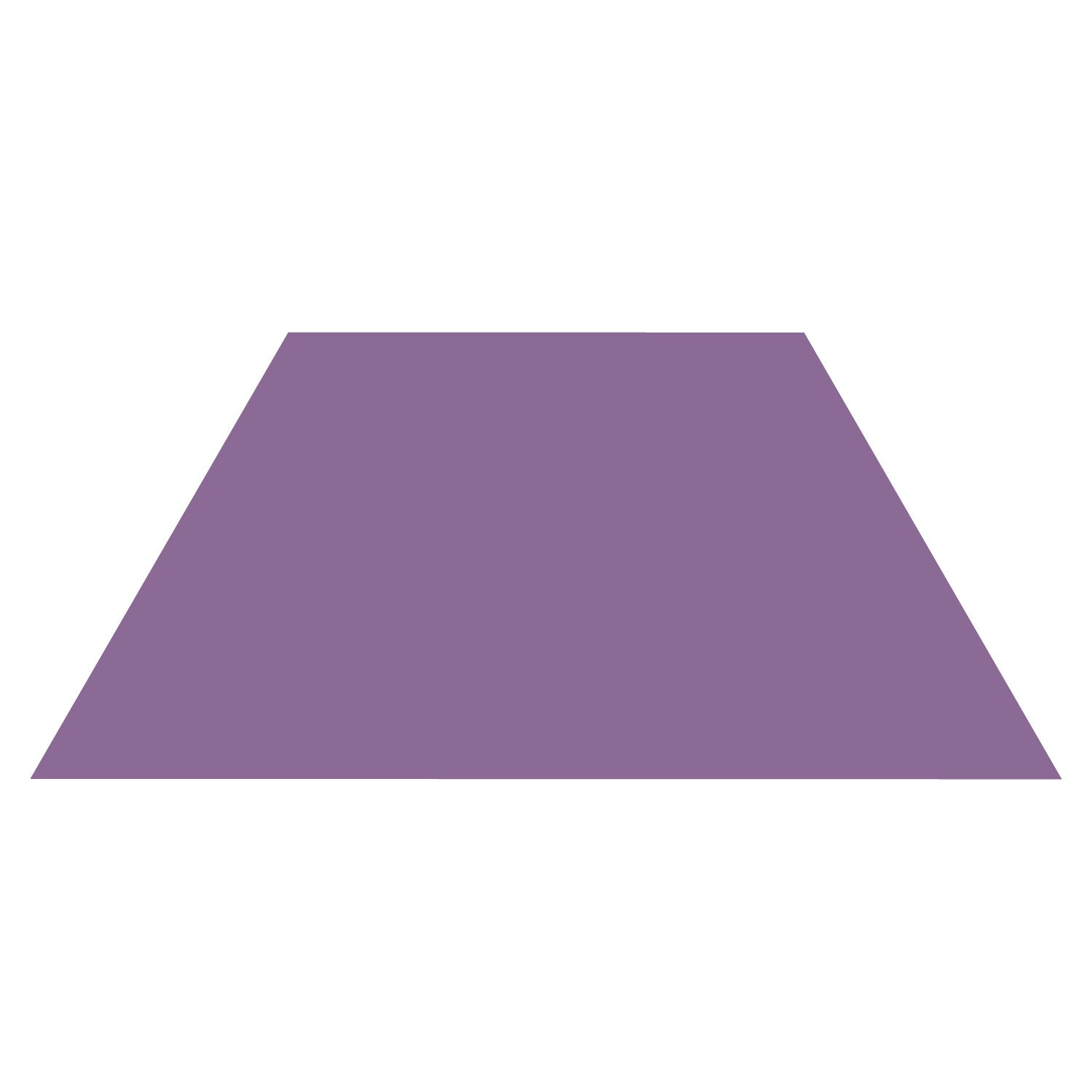100

57 x 6

342

100

603 divided by 3

201

100
Round 26 to the nearest tens place.

30

100

10 x 10=

100

100

There were 34 kids in the cafeteria. 6 kids can sit at a table. How many tables were full?

5 tables

100

True or False

1/2 < 1/3

False

1/2 = 3/6

1/3 = 2/6

3/6 is greater than 2/6

100

Name this polygonSquare

200

38 x 9

342

200

2,898 divided 9

322

200

Round 240 to the nearest hundred

200

DAILY DOUBLE

200

200 x 2 =

400

200

What does the word sum mean in math?

200

Place in order from least to greatest

8/7       1/3      4/5

1/3              4/5             8/7

200

How many lines of symmetry is in this shape?1

300

73x5

365

300

750 divided by 6 is...

125

300

What is the value of the number 6 in the following number, 56,789?

6,000

300

50 x 30 =

1,500

300

Is the number 29 a prime or composite number?

Prime!
300

How can the fraction 3/7 be decomposed?

2/7 + 1/7 OR 1/7 + 1/7 + 1/7

300

The perimeter of an octagon is 168 centimeters. Each side of the octagon is the same length. What is the length of one side of the octagon?

21cm

168 divided by 8 = 21 cm

400

38 x 5

190

400

648 divided by 8

81

400

Round 27,449 to the nearest thousand.

27,000

400

700 x 30

21,000

400

What is the answer called in a multiplication problem?

product

400

Solve

6/10 - 2/10 =

4/10

400

A room has a length of 10 square inches and a width of 7 square inches. What is the area of this room?

70 square inches.

500

24x10

240

500

250 divided by 5

50

500

Round 402,756 to the nearest ten thousand.

400,000

500

2000 x 8

16,000

500

What is the difference between a multiple and factors?

Multiples are the repeated addition of a number.

Factors are all the ways of multiplying to get a specific number.

500

solve

2/7 + ___ = 5/7

3/7

500

A garden has a length of 25 inches and a width of 5 inches. What is the perimeter of the garden?

60 inches.

600
3,556 x 6

21,336

600

5,412 divided by 7

773 R 1

600

What is the name of an angle that has a degree of 67?

Acute Angle

600

60,000 x 7=

420,000

600

7,239 divided by 4

1809 Remainder 3

600

Solve

2  1/4 + 2  1/3

4  7/12

600

Mr. Diaz wants to put a fence around his rectangular-shaped yard. The width of the yard is 65 feet. The length is 9 feet. What is the area of space inside the fence?

585 feet

Click to zoom